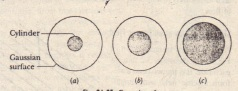# QUESTIONS Gauss Law

QUESTIONS Gauss Law

A sudace has the area vector A = (2l + 31) m2 What is the flux of an electric field through it if the field is (a) E = 4i N/C and (b) E = 4k N/C What is J dA for (a) a square of edge length a (b) a circle of radius r and (c) the curved surface of a cylinder of length hand radius r a full Gaussian surface encloses two of the four positively charged particles (a) Which of the particles contribute to the electric field at point P on the surface (b) Which net flux
of electric field through the surface is greater (if either) that due to ql and q2 or that due to all four charges in cross section a central metal ball, two spherical metal shells, and three spherical Gaussian surfaces of ra- Shell
dii R 2R and 3R all with the same center The uniform charges on the three objects are  Q smaller shell 3Q larger shell 5Q Rank the Gaussian surfaces
according to the magnitude of the electric field at any point on the surface greatest first Gaussian surfaces, each half-submerged in a large thick metal plate with a uniform surface charge density Gaussian surface 51 is the tallest and has the smallest square end caps; surface 53 is shortest and has the largest square end caps and has intermediate values Rank the surfaces according to (a) the charge they enclose (b) the magnitude of the electric field at points on their top end cap (c) the net electric flux through that top end cap and (d) the net electric flux through their bottom end cap greatest first.shows in cross section three cylinders each of
uniform charge Q Concentric with each cylinder is a cylindrical
Gaussian surface all three with the same radius Rank the Gaussian
surfaces according to the electric field at any point on the surface
greatest first.7. Three infinite nonconducting sheets, with uniform surface charge densities a 2iT and 3iT are arranged to be parallel like the two sheets in What is their order from left to right if the electric field E produced by the arrangement has magnitude E = 0 in one region and E = 2iT/eo in another region 8. A small charged ball lies within the hollow of a metallic spherical
shell of radius R. Here, for three situations  are the net charges on the ball and shell. respectively (I) +4q. 0; (2) -6q, + IOq (3) +16q, -12q. Rank the situations according to the charge on (a) the inner surface of the shell and (b) the outer surface. most positive first 9. Rank the situations of Question 8 according to the magnitude of the electric field (a) halfway through the shell and (b) at a point 2R from the center of the shell. greatest first. 10 shows four spheres, each with charge Q uniformly distributed through its volume. (a) Rank the spheres according to their volume charge density, greatest first. The also shows a point P for each sphere, all at the same distance from the center of the sphere (b) Rank the spheres according to the magnitude of the electric field they produce at point P greatest first.